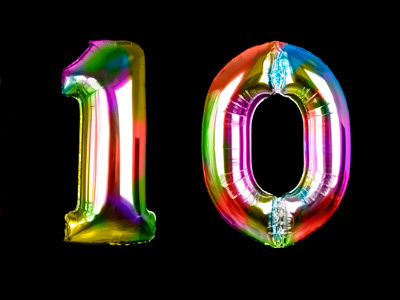Decimal numbers are fractions represented as tenths.

# Decimal Numbers 2 (Easy)

This is the second of our easy quizzes on decimal numbers. It may sound complicated, but the word ‘decimal’ simply means using tenths, hundredths, thousandths etc. to represent fractions. Tens, hundreds and thousands are shown before the decimal point, whilst tenths, hundredths and thousandths are shown after it.

Decimal numbers are easy, once you get the hang of them! Let’s take some fractions: a half, a quarter and a third. To represent these as decimals, simply divide the number at the top of the fraction by the number at the bottom. So, a half = 1 ÷ 2 = 0.5, a quarter = 1 ÷ 4 = 0.25 and a third = 1 ÷ 3 = 0.333.

Once you’ve mastered this quiz, and the other easy ones on decimal numbers, then why not move up a level to our medium quizzes? The more you practice using decimals, the sooner you will be confident with them.

1.
What are the place positions of the numbers in the decimal parts of the number 987.654?
4 is in the tenths place position, 5 is in the hundredths place position, 6 is in the thousandths place position
6 is in the tenths place position, 5 is in the hundredths place position, 4 is in the thousandths place position
4 is in the tenths place position, 6 is in the hundredths place position, 5 is in the thousandths place position
6 is in the tenths place position, 4 is in the hundredths place position, 5 is in the thousandths place position
TIP:
The first number to the right of the dp is in the tenths place position, e.g. 6 in 987.654
The second number to the right of the dp is in the hundredths place position, e.g. 5 in 987.654
The third number to the right of the dp is in the thousandths place position, e.g. 4 in 987.654
2.
What is three-quarters represented as a decimal number?
0.25
0.5
0.75
0.8
One-quarter is 0.25 so three-quarters is 0.25 + 0.25 + 0.25 = 0.75
3.
What is the value of the 1 in the number 654.321?
10 thousandths
1 thousandth
1 hundredth
1 tenth
Ten thousandths are the same as one-hundredth, and would be shown in decimal as 0.01
4.
What is one-quarter represented as a decimal number?
0.25
0.4
0.5
0.2
100 ÷ 4 = 25, so 1 ÷ 4 = 0.25
5.
What is the value of the 7 in the number 936.274?
7 tenths
7 hundredths
7 thousandths
1 seventh
Decimal numbers are in columns just like ordinary numbers - but backwards! Thousands, hundreds, tens, ones, tenths, hundredths, thousandths
6.
What is the value of the 4 in the number 234.567?
4 thousandths
4 hundredths
4 tenths
4
There is no 'ones' column after the decimal point - it's tenths first, then hundredths, thousandths etc. But the 4 in 234.567 comes before the dp.
7.
What are the place positions of the numbers in the decimal parts of the number 0.468?
8 is in the tenths place position, 6 is in the hundredths place position, 4 is in the thousandths place position
0 is in the tenths place position, 4 is in the hundredths place position, 6 is in the thousandths place position
4 is in the tenths place position, 6 is in the hundredths place position, nothing is in the thousandths place position
4 is in the tenths place position, 6 is in the hundredths place position, 8 is in the thousandths place position
There is no 'ones' column after the decimal point - it's tenths first, then hundredths, thousandths etc.
8.
What is the value of the 9 in the number 756.098?
1 ninth
9 tenths
9 hundredths
9 thousandths
TIP:
The first number to the right of the dp is in the tenths place position, e.g. 0 in 756.098
The second number to the right of the dp is in the hundredths place position, e.g. 9 in 756.098
The third number to the right of the dp is in the thousandths place position, e.g. 8 in 756.098
9.
What is two-quarters represented as a decimal number?
0.125
0.25
0.5
0.75
Two-quarters = one-half. One-half as a decimal is 1 ÷ 2 = 0.5
10.
What are the place positions of the numbers in the decimal parts of the number 12.345?
3 is in the tenths place position, 4 is in the hundredths place position, 5 is in the thousandths place position
5 is in the tenths place position, 4 is in the hundredths place position, 3 is in the thousandths place position
4 is in the tenths place position, 5 is in the hundredths place position, 3 is in the thousandths place position
3 is in the tenths place position, 5 is in the hundredths place position, 4 is in the thousandths place position
Decimal numbers are in columns just like ordinary numbers - but backwards! Thousands, hundreds, tens, ones, tenths, hundredths, thousandths
Author:  Frank Evans